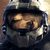0

# execution time not proportional to array length

dattorro 1 week ago updated by Pavel Holoborodko 3 days ago

Pavel,

Doubling array size quadruples execution time.

I'm not asking for help debugging code; this is something you need to see.

Look at it under the Matlab Profiler.

Jon

______________________________________________________________________

%MP Toolbox timing anomaly: twice the array size takes four times the execution time

clearvars

Fs = mp('48000');

freq = mp('997');

NumSecs = mp('1');  %record duration in seconds

%%%%%%%%%%%%%%%%%%%%%%%%%%%%%%%%%%%%%%%%%%%%%%%%%%%%%%%%%%%%%

N = NumSecs*Fs;

disp(['generating ' num2str(double(NumSecs)) '-second D/A signal'])

y1 = zeros(N,1,'mp');

y2 = zeros(N,1,'mp');

cosom = cos(mp('2*pi')*freq/Fs);

tic

for n=2:N  %for loop necessary in production code

y1(n) = cosom*y1(n-1);  %7.5 seconds in Matlab Profiler, AMD 3995WX CPU Windows 10

y2(n) = cosom*y2(n-1);  %7.5 seconds in Matlab Profiler, AMD 3995WX CPU Windows 10

end

toc

%%%%%%%%%%%%%%%%%%%%%%%%%%%%%%%%%%%%%%%%%%%%%%%%%%%%%%%%%%%%%

%%% DOUBLING number of array elements takes FOUR times the previous execution time %%%

NumSecs = mp('2');  %record duration in seconds

%%%%%%%%%%%%%%%%% EXACT DUPLICATE (cut & paste) of code block above %%%%%%%%%%%%%%%%

N = NumSecs*Fs;

disp(['generating ' num2str(double(NumSecs)) '-second D/A signal'])

y1 = zeros(N,1,'mp');

y2 = zeros(N,1,'mp');

cosom = cos(mp('2*pi')*freq/Fs);

tic

for n=2:N  %for loop necessary in production code

y1(n) = cosom*y1(n-1);  %37 seconds in Matlab Profiler, AMD 3995WX CPU Windows 10

y2(n) = cosom*y2(n-1);  %37 seconds in Matlab Profiler, AMD 3995WX CPU Windows 10

end

toc

%%%%%%%%%%%%%%%%%%%%%%%%%%%%%%%%%%%%%%%%%%%%%%%%%%%%%%%%%%%%%Jon,

Thank you very much for the excellent question.

MATLAB makes this operation slow for third-party toolboxes. In short, it requires the toolbox to make a copy of the whole array, even if only one element is requested (MEX API restrictions).

That is why we always suggest vectorizing code as much as possible (avoid loops and process data as a whole).
However application of IIR filter cannot be easily vectorized. We have been planning to add it to the toolbox core with all possible optimizations for a long time. Your request motivated us to prioritize this. Thanks!

Please download a new version of the toolbox (4.9.0.14753) from our website. Among other changes it includes the function 'filter' which implements IIR filter.

```tic
r1 = filter(, [1 -cosom], y1, [y1(1)]);
r2 = filter(, [1 -cosom], y2, [y2(1)]);
toc```

However, if you need to apply same filter on several arrays simultaneously, we would suggest to process them altogether:

```y = [y1 y2];
tic
r = filter(, [1 -cosom], y, [y1(1) y2(1)]);
toc```

This will allow the toolbox process each array (column) in parallel, using different CPU cores.